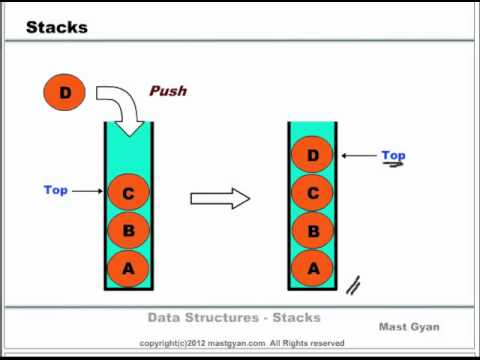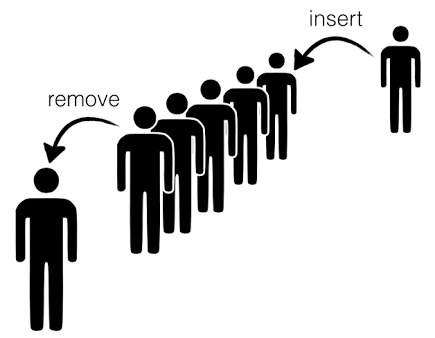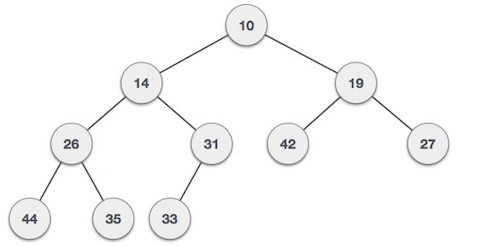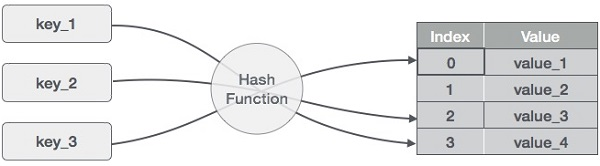StackStacks are dynamic data structures that follow the Last Out (LIFO) principle. The last item to be inserted a stack is the first one to be deleted from it.

For example, you have a stack of trays on a table. The tray at the top of the stack is the first item to be moved if you require a tray from that stack.

Inserting and deleting elements

have restrictions on the insertion and deletion of elements. Elements can be inserted or deleted only from one end of the stack i.e. the top. The element at the top is called the top element. The operations of inserting and deleting elements are called push) and pop) respectively.

When the top element of a stack is deleted, if the stack remains non-empty, then the element just below the previous top element becomes the new top element of the stack.

For example, in the stack of trays, if you take the tray on the top and do not replace it, then the second tray automatically becomes the top element (tray) of that stack.

QueueQueues are data structures that follow the First In First Out (FIFO) i.e. first element that is added to the queue is the first one to be removed.

Elements are always added to the back and removed from the front. Think of it as a line of people waiting for a bus. The person who is at the beginning of the line is the first one to enter the bus.

Variables used

• ]: Array in which queue is simulated
• : Maximum number of elements that can be stored in a queue]
• : Points at the index where the next deletion will be performed
• : Points at the index where the next insertion will be performed

Functions supported

Queues support the following fundamental functions:

Enqueue

function adds an element to the back of the queue.

Dequeue

function removes the element from the front of the queue.

Heaps

is a special case of balanced binary tree data structure where the root-node key is compared with its children and arranged accordingly. If α has child node β then −

key(α) ≥ key(β)

As the value of parent is greater than that of , this property generates Max Heap. Based on this criteria, a heap can be of two types −

For Input → 35 33 42 10 14 19 27 44 26 31

Min-Heap − Where the value of the root node is less than or equal to either of its children.Max-Heap − Where the value of the root node is greater than or equal to either of its children.Both trees are constructed using the same input and order of arrival.

Hash Table:

Hash Table is a data structure which stores data in an associative manner. In a hash table, data is stored in an array format, where each data value has its own unique index value. Access of data becomes very fast if we know the index of the desired data.

Thus, it becomes a data structure in which insertion and search operations are very fast irrespective of the size of the data. Hash Table uses an array as a storage medium and uses to generate an index where an element is to be inserted or is to be located from.

Hashing is a technique to convert a range of key values into a range of indexes of an array. We’re going to use to get a range of key values. Consider an example of of size 20, and the following items are to be stored. Item in the (keyvalue) format.

• (1,20)
• (2,70)
• (42,80)
• (4,25)
• (12,44)
• (14,32)
• (17,11)
• (13,78)
• (37,98)Sr.No. Key Hash Array Index 1 1 1 % 20 = 1 1 2 2 2 % 20 = 2 2 3 42 42 % 20 = 2 2 4 4 4 % 20 = 4 4 5 12 12 % 20 = 12 12 6 14 14 % 20 = 14 14 7 17 17 % 20 = 17 17 8 13 13 % 20 = 13 13 9 37 37 % 20 = 17 17

Whenever an element is to be searched, compute the hash code of the key and locate the element using that hash code as in the array. Use linear probing to get the element ahead if the element is not found the computed hash code.